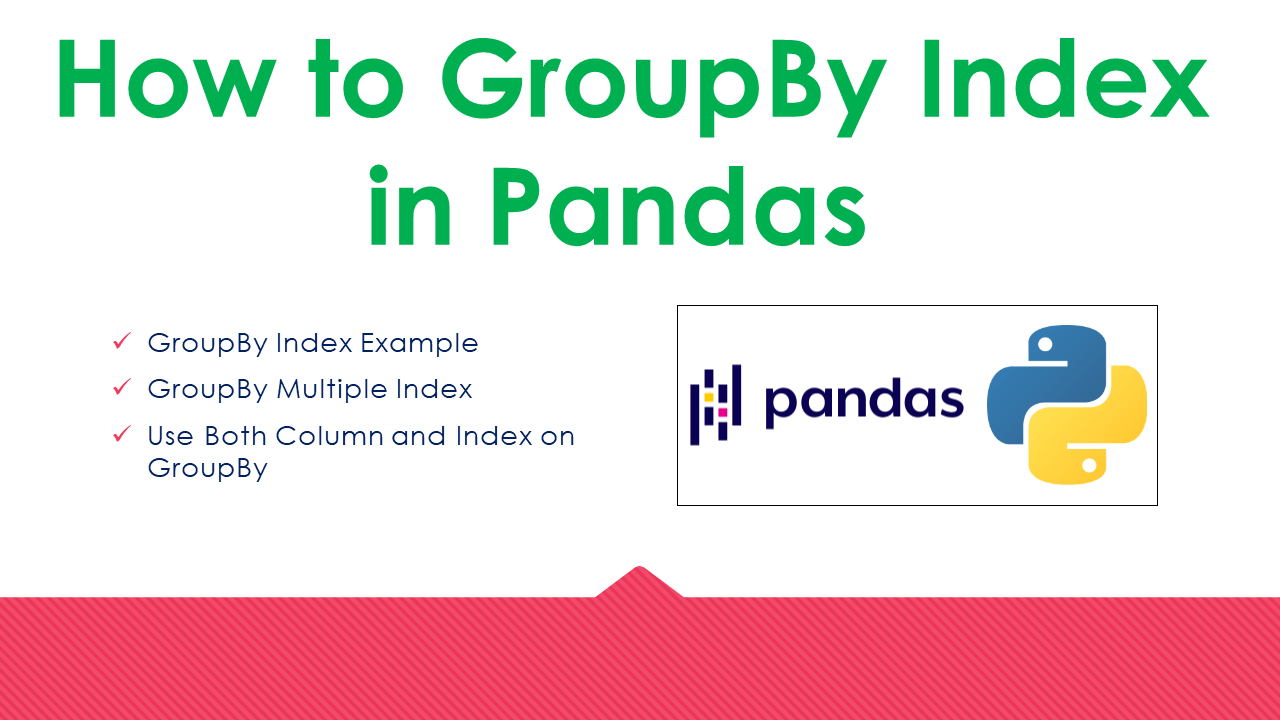# Pandas – How to GroupBy Index?

How to perform groupby index in pandas? Pass index name of the DataFrame as a parameter to `groupby()` function to group rows on an index. DataFrame.groupby() function takes string or list as a param to specify the group columns or index. When using the list you can also use the combination of index and columns.

In this article, I will explain how to perform group-by on a single index, multiple indexes, a combination of column and index with examples.

## 1. Quick Examples of Performing GroupBy on Index

Following are quick examples of how to perform group by on index field.

``````
# Below are quick examples

# Create DataFrame
df = pd.DataFrame(technologies)
# Set Index to DataFrame
df.set_index(['Courses','Fee'], inplace=True)
print(df)

# Groupby Index
result = df.groupby('Courses').sum()
print(result)

# Groupby Multiple Index
result = df.groupby(['Courses','Fee']).sum()
print(result)

# Groupby Column & Index
result = df.groupby(['Courses','Duration']).sum()
print(result)

``````

Let’s create a pandas DataFrame from the Dict object and explore the above examples.

``````
import pandas as pd
technologies = {
'Fee' :[20000,25000,26000,22000,25000,20000,35000],
'Duration':['30day','40days','35days','40days','60days','60days','70days'],
'Discount':[1000,2300,1200,2500,2000,2000,3000]
}

df = pd.DataFrame(technologies)
df.set_index(['Courses','Fee'], inplace=True)
print(df)
``````

Yields below output. As you notice in the above example, I have used DataFrame.set_index() to set the multiple columns as Index. I will use these two indexes to group rows.

``````
Duration  Discount
Courses Fee
Spark   20000    30day      1000
PySpark 25000   40days      2300
Python  22000   40days      2500
PySpark 25000   60days      2000
Spark   20000   60days      2000
35000   70days      3000
``````

## 2. GroupBy Index Example

DataFrame.gorupby() accepts string or list of column or index names to perform group in pandas DataFrame. The index name is required to perform, If you don’t have it, set the name to index by using `DataFrame.index.name = 'index-name'`.

``````
# Groupby Index
result = df.groupby('Courses').sum()
print(result)
``````

Yields below output.

``````
Discount
Courses
PySpark      4300
Python       2500
Spark        6000
``````

## 3. GroupBy Multiple Index

Now let’s see how to group by multiple index fields at the same time, to do so pass all index names as a list. The below example groups rows by `Courses` and `Fee` index.

``````
# Groupby Multiple Index
result = df.groupby(['Courses','Fee']).sum()
print(result)
``````

Yields below output.

``````
Discount
Courses Fee
PySpark 25000      4300
Python  22000      2500
Spark   20000      3000
35000      3000
``````

## 4. Use Both Column and Index on GroupBy

Sometimes you may also be required to do group by on column and index at the same time, the good thing about `groupby()` function is it accepts both at the same time.

``````
# Groupby Column & Index
result = df.groupby(['Courses','Duration']).sum()
print(result)
``````

Note that my DataFrame doesn’t have the right date to the group on `Courses` and `Duration` columns hence it listed all rows from DataFrame. But I hope you understand the concept of how to do it.

``````
Discount
Courses Duration
PySpark 40days        2300
60days        2000
Python  40days        2500
Spark   30day         1000
60days        2000
70days        3000
``````

## 5. Complete Example

``````
import pandas as pd
technologies = {
'Fee' :[20000,25000,26000,22000,25000,20000,35000],
'Duration':['30day','40days','35days','40days','60days','60days','70days'],
'Discount':[1000,2300,1200,2500,2000,2000,3000]
}

df = pd.DataFrame(technologies)
df.set_index(['Courses','Fee'], inplace=True)
print(df)

# Groupby Index
result = df.groupby('Courses').sum()
print(result)

# Groupby Multiple Index
result = df.groupby(['Courses','Fee']).sum()
print(result)

# Groupby Column & Index
result = df.groupby(['Courses','Duration']).sum()
print(result)
``````

### Conclusion

In this article, you have learned how to perform group by on a single index, multiple indexes, and a combination of column and index using pandas groupby() function.

References

### NNK

SparkByExamples.com is a Big Data and Spark examples community page, all examples are simple and easy to understand and well tested in our development environment Read more ..